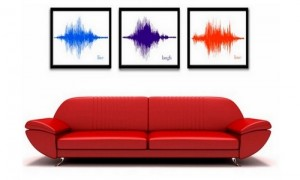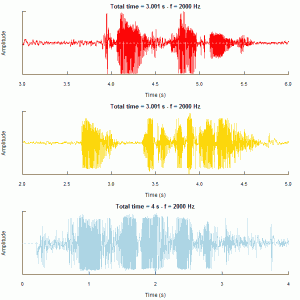# Mandelbrot Set

1.ggplot2的图形，循环迭代较多，运行确实很慢。

 ```1 2 3 4 5 6 7 8 9 10 11 12 13 14 15 16 17 18 19 20 21 22 23 24 25 26 27 28 29 30 31 32 33 34 35 36 37 38 39 ``` ```library(ggplot2) max_iter = 25 cl = colours() step = seq(-2, 0.8, by = 0.005) points = array(0, dim = c(length(step)^2, 3)) t = 0 for (a in step) { for (b in step + 0.6) { x = 0 y = 0 n = 0 dist = 0 while (n < max_iter & dist < 4) { n = n + 1 newx = a + x^2 - y^2 newy = b + 2 * x * y dist = newx^2 + newy^2 x = newx y = newy }   if (dist < 4) { color = 24 } else { color = n * floor(length(cl)/max_iter) }   t = t + 1 points[t, ] = c(a, b, color) } } df = as.data.frame(points) ggplot(data = df, aes(V1, V2, color = cl[V3])) + geom_point(size = 0.5) + opts(panel.background = theme_blank(), panel.grid.major = theme_blank(), panel.grid.minor = theme_blank(), axis.ticks = theme_blank(), axis.text.x = theme_blank(), axis.text.y = theme_blank(), axis.title.x = theme_blank(), axis.title.y = theme_blank(), legend.position = "none")```2. 动态看图形变化，此转于此处，代码简洁易理解。不过代码做出来的图与原网页展示的图形不同，颜色怎么调都没有他们的漂亮，主要是迭代次数还不够，也不够精细，直接看原链接的吧，搭配颜色是个细致活，伤脑细胞。

# 爱的声音```1 2 3 4 5 6 7 8 9 10 11 12 ``` ```library(seewave) library(tuneR) woaini = readMP3("我爱你.mp3") woaini1=resamp(woaini, g = 2000) muqinjie = readMP3("母亲节快乐.mp3") muqinjie1 = resamp(muqinjie, g = 2000) iloveyou = readMP3("i_love_you.mp3") iloveyou1 = resamp(iloveyou, g = 2000) op = par(mfrow = c(3,1),mar = c(4.5,4,2,2)) oscillo(woaini1, f = 2000, from = 3, to = 6,cexlab = 0.75,colwave = "red",title = "妈-我爱你") oscillo(muqinjie1, f = 2000, from = 2, to = 5,cexlab = 0.75,colwave="gold",title = "妈-母亲节快乐") oscillo(iloveyou1, f = 2000, from = 0, to = 4,cexlab = 0.75,colwave="lightblue",title = "i love you so much")```• 最上是我用家乡话（信阳罗山话）说的：“妈，我爱你！”（罗山话中，“你”发音类似于“恩”，故振幅小）
• 中间一幅依然用家乡话：“妈，母亲节快乐！”（录得不好，貌似有杂音）
• 最下面的那副是英文：“I love you so much！”